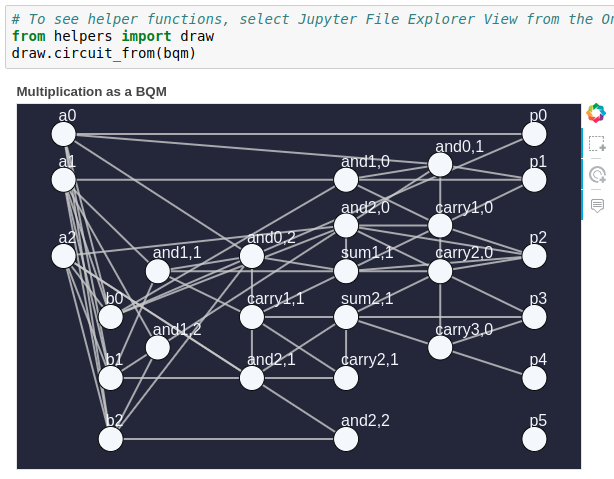# How to create a CSP for a 4-bit multiplication circuit.

Hello,

I''ve run the Jupyter Notebook demo for Factoring with the D-Wave System, nice :-)

Question: I wanted to factor a larger number, ex: 62, you then need a 4 bit input. The code in "Factoring on the Quantum Computer" section, second cell is given for a 3-bit input:

`csp = dbc.factories.multiplication_circuit(3)# Print one of the CSP's constraints, the gates that constitute 3-bit binary multiplicationprint(next(iter(csp.constraints)))`

So I thought I just had to change the 3 for a 4 on the first line, but the output of the cell remains:

`Constraint.from_configurations(frozenset({(1, 0, 0), (1, 1, 1), (0, 1, 0), (0, 0, 0)}), ('a0', 'b0', 'p0'), Vartype.BINARY, name='AND(a0, b0) = p0')Which looks like a 3-bit input to me.How can we change to a 4-bit input ?`

• Hi Hugues,

Try this for the print statement:

`# print(next(iter(csp.constraints))) # use the loop below instead of a single printfor c in iter(csp.constraints):    print(c)`

So i changed the cell to :

`csp = dbc.factories.multiplication_circuit(4)for c in iter(csp.constraints): print(c)`

Which gives me an output that is longer than before, which makes sense. But when I run the next 2 cells, the BQM i get (below) seems to be still for an input of 3bits x 3 bits, no ? Is there something more that I need to change to use 4 bits x 4 bits inputs ?• I tried it with different sizes of n, and it looked like draw.circuit_from() is hard coded to display a 3-bit multiplier. Looking through the source code confirmed this: /demos/factoring/helpers/draw.py

circuit_from() is calling circuit_layout, which has hard-coded position values for rendering an image.

The next thing to change would be changing the fixed variables:

`# p_vars = ['p0', 'p1', 'p2', 'p3', 'p4', 'p5'] # this was for a 6 bit numberp_vars = ['p0', 'p1', 'p2', 'p3', 'p4', 'p5', 'p6', 'p7'] # we need 8 bits for a 4x4 multiply`

Finally, we hit a snag with the embedding. The embedding for the example in this notebook was hard-coded and put in a helper file: /demos/factoring/helpers/embedding.py

This is problematic because a 4x4 multiplier uses variables not defined in the hard-coded embedding. (E.g., and0,3.)

All that said, this particular Jupyter notebook (for factoring) is probably not suitable for arbitrary enhancement.

• The last section of the factoring notebook "Minor-Embedding" shows how to create your own minor embeddings with the minorminer tool.

• I do

1. modify .  bP = "{:08b}".format(P)

2. modify   csp = dbc.factories.multiplication_circuit(4)

3. modify   p_vars = ['p0', 'p1', 'p2', 'p3', 'p4', 'p5', 'p6', 'p7']

4. use embedding = minorminer.find_embedding(bqm.quadratic, target_edgelist)

5. change to_base_ten() function too

and final got

`Given integer P=33, found factors a=15 and b=15`

anyone have good code example for 4-bit ?   Thanks!!!

•

Take a look at the the function multiplcation_circuit() in the file:

https://github.com/dwavesystems/dwavebinarycsp/blob/master/dwavebinarycsp/factories/csp/circuits.py

There is some documentation there for generic usage that you can probably use to factor. Copying from the docs in that file:

`import dwavebinarycspfrom dwavebinarycsp.factories.csp.circuits import multiplication_circuitimport nealcsp = multiplication_circuit(3)bqm = dwavebinarycsp.stitch(csp)bqm.fix_variable('a0', 1); bqm.fix_variable('a1', 0); bqm.fix_variable('a2', 1)bqm.fix_variable('b0', 1); bqm.fix_variable('b1', 1); bqm.fix_variable('b2', 0)sampler = neal.SimulatedAnnealingSampler()response = sampler.sample(bqm)p = next(response.samples(n=1, sorted_by='energy'))print(p['p0'], p['p1'], p['p2'], p['p3'], p['p4'], p['p5']) # doctest: +SKIP 1 1 1 1 0 0`

And here I changed the code to factor the number 33:

`import dwavebinarycspfrom dwavebinarycsp.factories.csp.circuits import multiplication_circuitimport nealcsp = multiplication_circuit(3)bqm = dwavebinarycsp.stitch(csp)# bqm.fix_variable('a0', 1); bqm.fix_variable('a1', 0); bqm.fix_variable('a2', 1) # bqm.fix_variable('b0', 1); bqm.fix_variable('b1', 1); bqm.fix_variable('b2', 0) bqm.fix_variable('p0', 1);bqm.fix_variable('p1', 0);bqm.fix_variable('p2', 0);bqm.fix_variable('p3', 0);bqm.fix_variable('p4', 0);bqm.fix_variable('p5', 1);sampler = neal.SimulatedAnnealingSampler()response = sampler.sample(bqm)p = next(response.samples(n=1, sorted_by='energy'))# print(p['p0'], p['p1'], p['p2'], p['p3'], p['p4'], p['p5']) print(p['a0'], p['a1'], p['a2'], p['b0'], p['b1'], p['b2'])`

This code should be more straightforward to extend.

• Thanks!!!!  I got them.

After two times of 10000 #num_reads, I success factoring 33

`Given integer P=33, found factors a=11 and b=3`

And , I  use the 4 x 4 circuits because  a=11 need 4 bits ,  3 bits circuit only can hold 0-7.